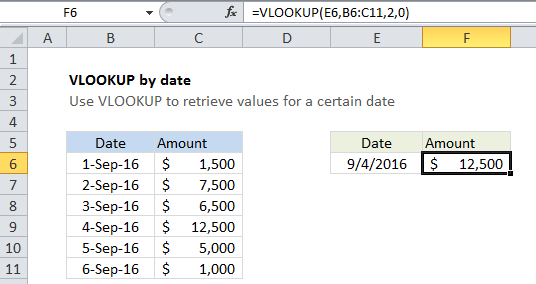## Excel Office

Excel How Tos, Tutorials, Tips & Tricks, Shortcuts

# VLOOKUP by date in Excel

This tutorial shows how to calculate VLOOKUP by date in Excel using the example below;

## Formula

`=VLOOKUP(date,table,col,FALSE)`## Explanation

To retrieve a value on a specific date from a table, you can use the VLOOKUP function.

In the example shown, the formula in F6 is:

`=VLOOKUP(E6,B6:C11,2,0)`

### How this formula works

This is a standard VLOOKUP formula. It requires a table with lookup values (in this case, dates) to the left of the values being retrieved.

Worked Example:   Map inputs to arbitrary values in Excel

The lookup value comes from cell E6, which must be a valid date. The table array is the range B6:C11, and the column index is 2, since the amounts are in the second column of the table. Finally, zero is provided for the final argument to force an exact match.

Worked Example:   Win loss points calculation in Excel

The VLOOKUP function locates the date value for Sept 4, and returns the value at the same row in the second column: 12,500.

Worked Example:   Get first text value in a list in Excel

Note: the lookup value and the date values in the table must be valid Excel dates.